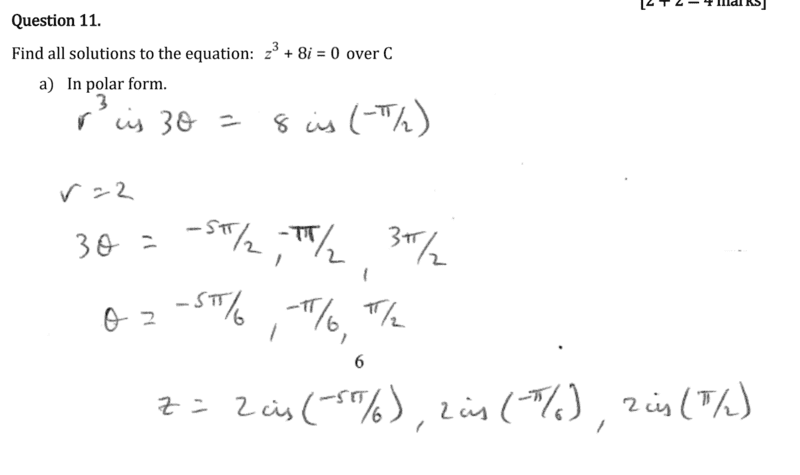# How do you know how many solutions there are for z?

Darkmisc
Homework Statement:
z^3+8i=0
Relevant Equations:
r^3cis3t = 8cis(-pi/2)
Hi everyone

How do you know how many solutions z has
a) in this problem
b) in general?

I understand that they are rotating 2 pi from (-pi/2) in both directions to get the other two solutions. Should this be done in all problems?
Is it simply a coincidence that there are three solutions when z is in the third power?

If the problem needed to be solved for z^4, would there be four solutions? Or only three (again by rotating 2 pi in both directions)?

Thanks#### Attachments

•Delta2

••